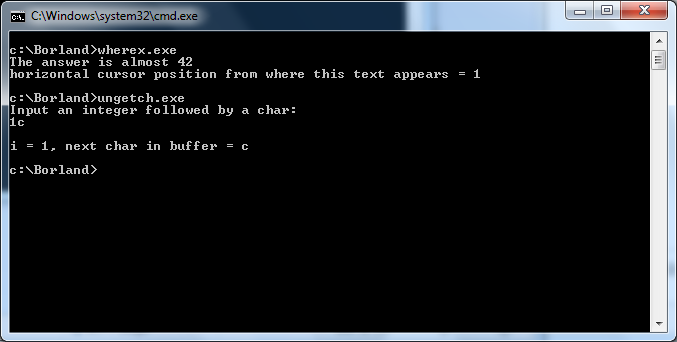### Programming Reference/Librarys

Q&A is closed

c:conio.h:ungetch# ungetch

`int ungetch(int ch);`

### description of ungetch

Pushes a character back into the keyboard buffer.

return
Successful execution ungetch returns the character ch.
If an error occurs, the function returns EOF.

```#include <stdio.h>
#include <ctype.h>
#include <conio.h>

int main(void)
{
int i=0;
char ch;
puts("Input an integer followed by a char:");

while((ch = getche()) != EOF && isdigit(ch))
i = 10 * i + ch - 48;

if (ch != EOF)
ungetch(ch);
printf("\n\ni = %d, next char in buffer = %c\n", i, getch());
return 0;
}```

## output of ungetch c example

``` The answer is almost 42
horizontal cursor position from where this text appears = 1

c:\Borland>ungetch.exe
Input an integer followed by a char:
1c

i = 1, next char in buffer = c```